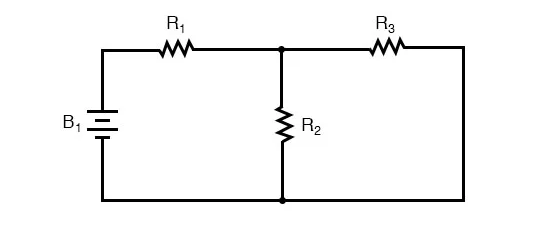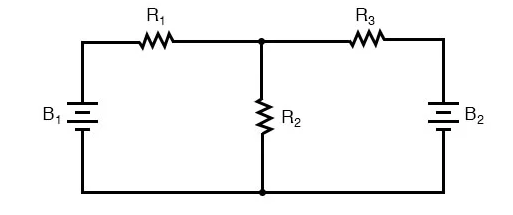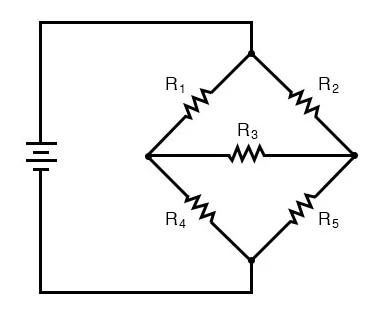Checkout JEE MAINS 2022 Question Paper Analysis : Checkout JEE MAINS 2022 Question Paper Analysis :

# Network Analysis

Network analysis is a process through which we calculate various electrical parameters of a circuit element connected in an electrical network. It uses mathematical tools to analyze a circuit. Engineers and technicians quite often encounter circuits that contain multiple sources of power or configurations that resist simplification by the series\parallel analysis techniques.  In the next few sections, let us learn to examine circuits to find various electrical parameters.

## Terminologies in Network Analysis

Here are a few important terminologies used in network analysis along with their definitions.

 Component – A device with two or more terminals into which the current may or may not flow Node – A point at which terminals of more than two components are joined. Branch – A component joining two nodes Mesh – A group of branches within a network joined so as to form a complete loop such that there is no other loop inside it. Port – Two terminals where the current into one is identical to the current out of the other.

## Examining a Simple Circuit

As an example, let us consider the below series-parallel circuit.To analyze the circuit, first one would have to find the equivalent of R2 and R3, then add the series resistor R1 to arrive at the total resistance. With the identified total resistance and the voltage of the battery, the total current can be calculated using Ohm’s Law.

## Inspecting a Complex Circuit

Now, let us consider a circuit with two batteries instead.The resistors R2 and R3 are no longer in parallel because B2 has been inserted into R3’s branch of the circuit. Upon closer inspection, you will notice that no two resistors are in series or parallel. This seemingly simple circuit, with just three resistors, cannot be reduced to a combination of simple series and simple parallel sections: it is something different altogether. However, this is not the only type of circuit defying series/parallel analysis:

### Unbalanced Bridge CircuitLet us consider the given bridge circuit for the sake of example and let us consider it to be unbalanced (in other words, the ratio R1/R4 is not equal to the ratio R2/R5). If it were balanced, then no current would flow across the resistor R3, and we could have considered it to be balanced and approached it as a series/parallel circuit. Resistors R1 and R4 are not in series because there is another path for the current to flow across, i.e, through R3. Similarly, R2 and R5 are not in series, for the same reason.

In the case of a two-battery circuit, there is no way to arrive at the total resistance due to the presence of two sources of voltage and current. In the case of an unbalanced bridge circuit, the total current splits into unknown proportions at the end of each bridge, so no further calculations can be carried out. So, when we are faced with multiple unknowns in a circuit, we use a mathematical process known as simultaneous equations or systems of equations. In this process, the multiple unknowns are solved by relating them to each other in the form of multiple equations. While solving multiple unknowns, we need to have the same number of equations as the same number of unknowns to be found to reach a solution.

## Frequently Asked Questions – FAQs

### What is meant by network analysis?

Network analysis is the process by which we find different parameters of a circuit component connected in an electrical circuit. It uses various mathematical methods to analyse a circuit.

### What are the main components of an electric circuit?

The main components of an electrical circuit are a conductive path, a source of electric power, and a load that requires electric power to function.

### What are the important terminologies used in network analysis?

The important terminologies used in network analysis are component, node, branch, mesh, and port.

### What are the three main types of circuits?

Parallel circuits, series circuits, and parallel-series circuits are the main types of circuits.

### What is a node?

A node is a point where terminals of multiple components are connected.

### What are the main elements used in an electrical circuit?

Resistors, capacitors, and inductors are the main elements used in an electrical circuit.

Test your Knowledge on Network Analysis